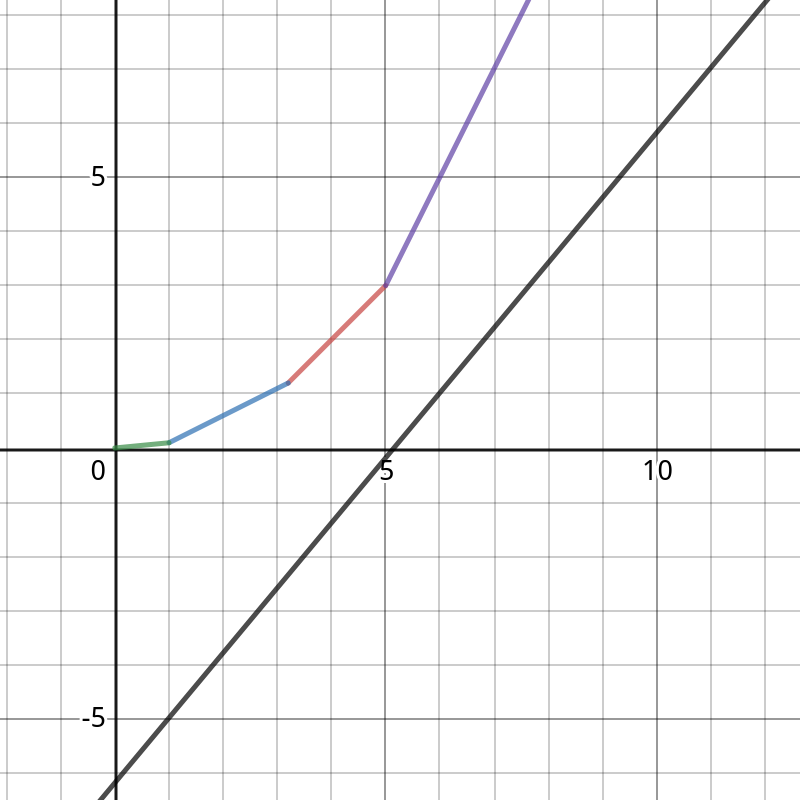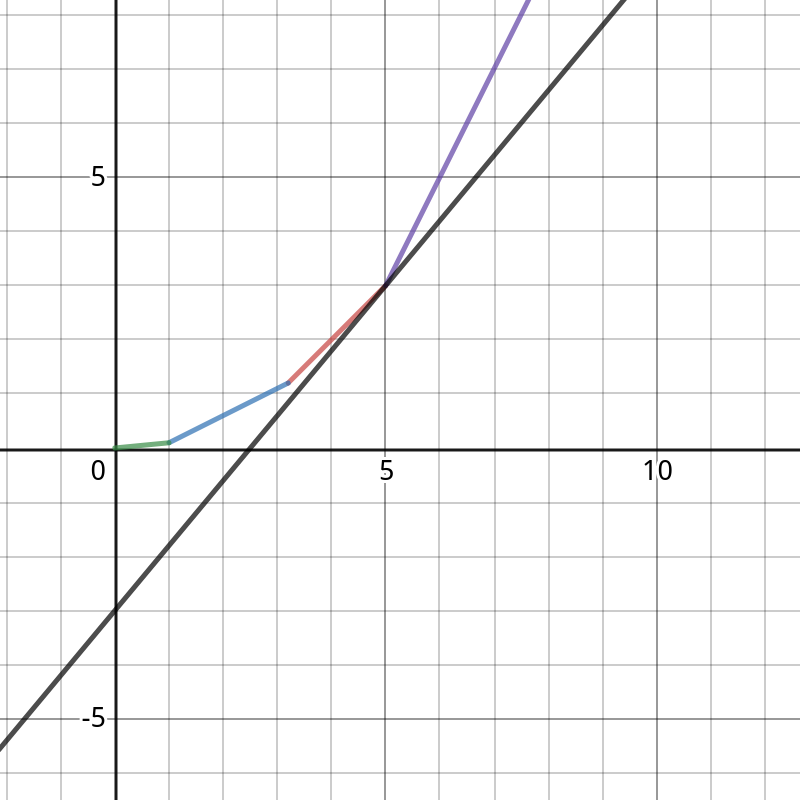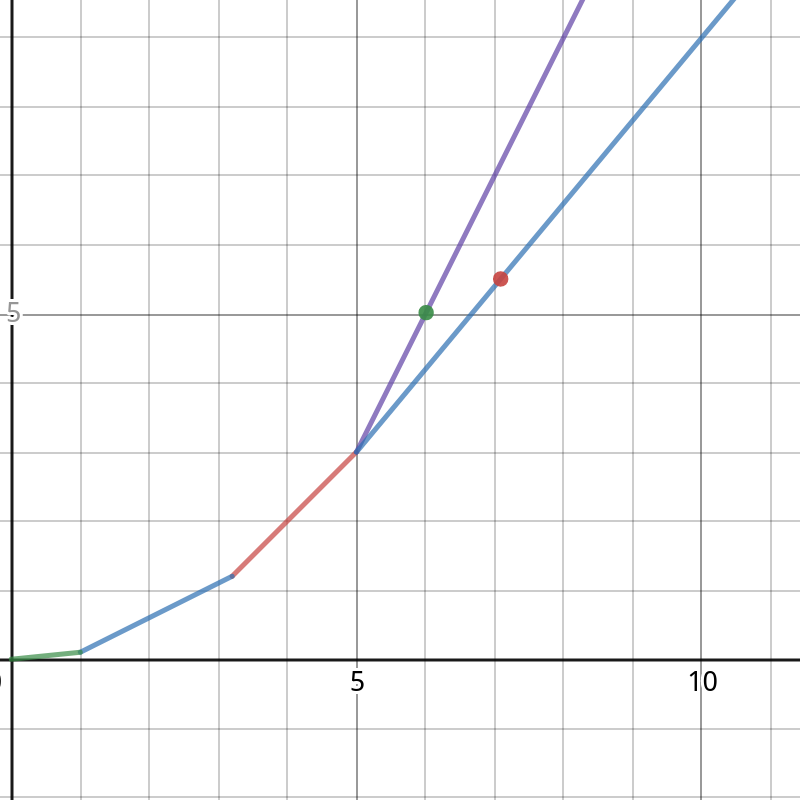# arrow_back斜率优化共7篇文章zc
2019-12-21 19:47:00

f_i为前i个任务的费用,t_i为前i个任务时间的总和,w_i为前i个任务费用的总和

 f_i=\min_{j=0}^{i-1}(f_j+s\times(w_n-w_j)+t_i\times(w_i-w_j)) 

 f_j+s\times(w_n-w_j)+t_i\times(w_i-w_j) \le f_k+s\times(w_n-w_k)+t_i\times(w_i-w_k) \\ t_i(w_k-w_j)\le f_k-f_j+s(w_j-w_k) \\ t_i\le \frac{f_k-f_j+s(w_j-w_k)}{w_k-w_j} \\ t_i+s\le \frac{f_k-f_j}{w_k-w_j}zc
2019-12-21 19:47:00

f_i表示前i块土地

 f_i=\min_{j=1}^{i-1}(f_j+maxw[j-1][i]\times maxh[j-1][i]) 

 f_i=\min_{j=1}^{i-1}(f_j+l[j+1]\times w[i]) 

 f_j+l[j+1]\times w[i]\le f_k+l[k+1]\times w[i] \\ w[i](l[j+1]-l[k+1])\le f_k-f_j \\ w[i]\le \frac{f_k-f_j}{l[j+1]-l[k+1]}zc
2019-12-21 19:47:00

f_i表示前i件玩具的制作费用

 f_i=\min_{j=1}^{i-1}(f[j]+(i-j-1+s_i-s_j-L)^2) 

a_i=s_i+i,b[i]=s_i+i+L+1

(都只与i有关)

x=b_j,y=f_j+{b_j}^2  y=2a_ix+(f_i-{a_i}^2) 

f_i含义转化为当上述直线经过点(x,y)(b_j,f_j+{b_j}^2)时,直线在y轴的截距上加上{a_i}^2

https://www.desmos.com/calculator/mqsdvzuaee1. while(s(q_h,q_{h+1})<2a_i)++h;
2. 当前队头的点最优,用这个点计算出f_i
3. while(s(q_{t-1},q_t)>s(q_{t-1},i))--t;
4. 队尾插入点izcmimi
2019-12-21 19:47:00

f_i表示前i个拆分后最大战斗力和

 f_i=\max_{j=1}^{i-1}(f_j+a(s_i-s_j)^2+b(s_i-s_j)+c) 

 f_j+a(s_i-s_j)^2+b(s_i-s_j)+c\ge f_k+a(s_i-s_k)^2+b(s_i-s_k)+c \\ 2as_i\le\frac{(f_k+a{s_k}^2-bs_k)-(f_j+a{s_j}^2-bs_j)}{s_k-s_j}zc
2019-12-21 19:47:00

f(i,k)表示将前i个分k次的分数

f_i为前i个,g_i为上一次dp的结果

 f_i=\max_{j=1}^{i-1}(g_j+s_j\times(s_i-s_j)) 

g_j+s_j\times(s_i-s_j)\ge g_k+s_k\times(s_i-s_k) 展开得

g_j+s_is_j-{s_j}^2\ge g_k+s_is_k-{s_k}^2 移项得 s_i(s_j-s_k) \ge {s_j}^2-{s_k}^2+g_k-g_j 整理得 s_i\ge \frac{({s_j}^2-g_j)-({s_k}^2-g_k)}{s_j-s_k}zc
2019-12-21 19:47:00zc
2019-12-21 19:47:00

f_i为前i个任务的费用,t_i为前i个任务时间的总和,w_i为前i个任务费用的总和

 f_i=\min_{j=0}^{i-1}(f_j+s\times(w_n-w_j)+t_i\times(w_i-w_j)) 

 f_j+s\times(w_n-w_j)+t_i\times(w_i-w_j) \le f_k+s\times(w_n-w_k)+t_i\times(w_i-w_k) \\ t_i(w_k-w_j)\le f_k-f_j+s(w_j-w_k) \\ t_i\le \frac{f_k-f_j+s(w_j-w_k)}{w_k-w_j} 

Search
search